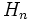Conjugate-dense sub-APS

A sub-APS$H$ of an APS of groups$(G,\Phi)$ is termed conjugate-dense if for every$n$,$H_n$ is conjugate-dense as a subgroup of$G_n$, i.e. for every$n$, every element of$G_n$ is conjugate (in$G_n$) to some element of$H_n$.